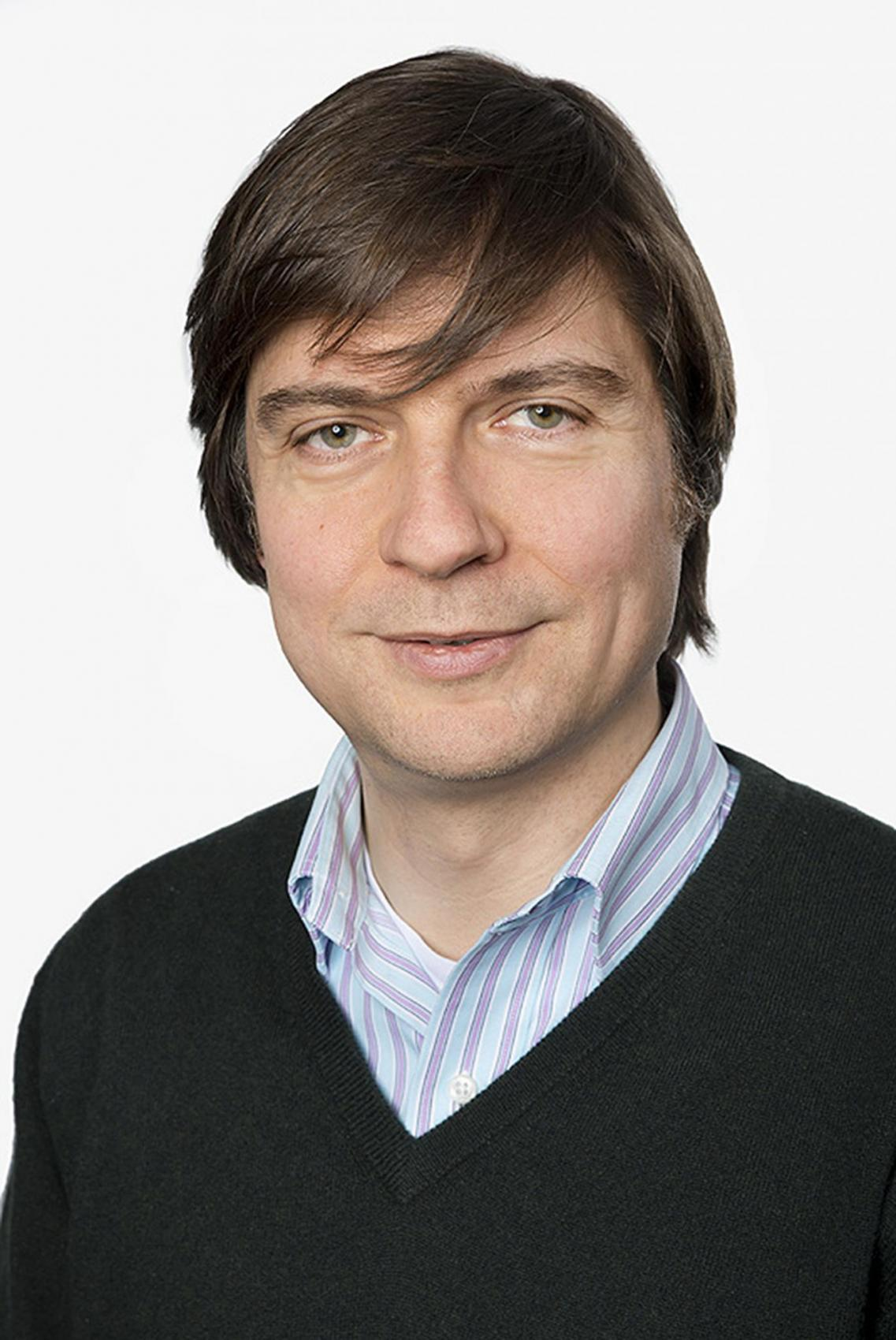distinguished lecture series presents

### Max Planck Institute for Mathematics in the Sciences

Mathematics

###### Visit

January 7, 2020 - January 9, 2020

MS 6627

# Website

## Randomness in Partial Differential Equations

###### abstracts
Quantitative stochastic homogenization – In the ﬁrst talk, the equations will be linear elliptic equations in divergence form, and the (quenched) randomness will reside in the coeﬃcient ﬁeld, reﬂecting an incomplete knowledge of a heterogeneous – say conducting – medium. If the statistics are stationary and ergodic, this random medium behaves on large scales like a homogeneous one – a qualitatively well-understood phenomenon known as stochastic homogenization. Making this connection fully quantitative is an area of recent activity; some representative results will be discussed. Key are suitable coordinates, their sensitivities w. r. t. the coeﬃcient ﬁeld, and elliptic regularity theory in form of “annealed” Calderón-Zygmund estimates.
Quasi-linear singular stochastic equations – In the second talk, the equations will be parabolic with a non-linearity in the leading-order term, and driven by thermal noise in the form of a random right hand side. The challenge comes from the fact that this “driver” is so rough that the nonlinearity requires a renormalization. We show that the tools and notions of regularity structures, devised for the semi-linear setting, also ﬁt this quasi-linear setting. Guided by considering an entire class of non-linearities, these tools provide a representation of the solution manifold, which is eﬃcient despite the low regularity of the solutions, and is stable in the presence of divergent terms. This representation by expansion is quantiﬁed through a kernel-free Schauder theory.
Matching Poisson to Lebesgue – In the third talk, we consider the problem of optimally matching the Poisson point process to the uniform distribution, in the thermodynamic regime. This problem in optimal transportation means solving the Monge-Ampère equation driven by a microscopically rough and random right hand side. Especially the two-dimensional case is subtle because of a logarithmic divergence in the macroscopic displacement, reminiscent of the Gaussian free ﬁeld. We mesoscopically capture this subtle behavior by a quantitative large-scale linearization of the Monge-Ampère equation through the Poisson equation. It relies on an entirely variational approach to the recent f-regularity theory for the Monge-Ampère equation.
###### Lecture 3
2300 Murphy Hall - Box 951438 - Los Angeles, CA 90095-1438 © 2018